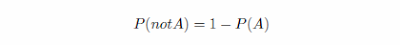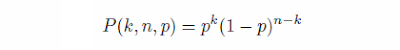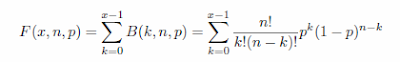## Apr 15, 2011

### Loot drop and other epic problems of probability theoryOne day my old friend called me and asked if I could give a hand in solving some school math problems (he was trying to tutor his nephew).

Among the problems was:
The factory produces some parts. Given that from every 60 parts 12 are defective, find the probability that from 10 parts at least two are defective.
Frankly, the solution did not occur immediately at all - it's been a while since the student years.

What did occur to me immediately, though, is that something is wrong with the way people are usually taught those things at school. There are often troubles remembering them afterwards. Why?..

Apparently, one of the reasons is that the problems which students are asked to solve are usually plain boring.

Why would I care about those "defective parts", indeed?...

Now, the funny part is that it has nothing to do with the mathematical apparatus at hand (which is the thing we are supposed to learn): being abstract as it is, it can be applied to many practical problems. And some of them might appear more interesting than others, depending on the context.

Two friends are playing World of Warcraft. They agreed to group for farming a boss, who drops a certain epic loot with a chance of 20%. What are the odds that after killing it 10 times both friends would get the desired epic gear?
See what I have done here?.. It is exactly the same problem. Except now it's better flavoured, and furthermore, it's actually a problem of practical interest to people playing WoW.

And something makes me feel that there are more young people playing WoW these days than those making defective parts at factories!

So, here's the (interactive) answer to the problem. Try entering different numbers and pressing the "Recompute" button (keep in mind that the code here does not attempt to be too robust with the boundary cases... also, values might be rounded, so 99.999999% will be shown as 100%, which is not correct):

With the loot drop chance of %, the odds of getting at least drop(s) after attempts are 0.012%.

It is computed by substituting values into a somewhat intimidating formula:Here n is the total amount of boss kills, p is the loot drop chance and x is the minimal desired amount of drops we can get.

The mentioned article uses particular case of this formula, when x is 1 (i.e. when we want to know the chance that the item drops at least once):Alright, but this is an answer, not a solution!

However, chances are that it is almost the implied solution to the original problem about the defective parts: the student is supposed to identify the Bernoulli process (AKA "repeated coin flipping"), remember that the number of successes in a given amount of trials has a binomial distribution, and use cumulative distribution function formula for that.

But this brings another problem with education: we are tought more about "what's" (facts and answers), than of "why's" (motivation and solutions). Resorting to just memoizing the formulas is not too reliable from the point of view of problem solving abilities.

So, let's try to unlearn all that again and start from the beginning.

#### Step 1

First, let's treat all our probabilities as being in the range [0, 1], not in percents (converting back and forward is just about multiplication/division by 100%).

One fundamental formula to consider is:Here P(A) means "probability that event A happens", and P(not A) means "probability that event A does not happen".
The chance of loot drop is 0.2 (which corresponds to 20%). What is the chance that there will be no loot drop?
The answer is 0.8 (1 - 0.2, using the formula).

#### Step 2

The chance that two independent events A and B would happen both is:The boss can drop the epic sword with 0.1 chance. What is the chance to kill the boss two times, and both times to get the sword?
The answer is 0.01 (0.1*0.1). We want both of the idependent events (sword drops) happen.

#### Step 3

One more basic formula from probability theory: if events A and B are mutually exclusive (either of them can happen, but not both together), then:The boss can drop one of two items: an epic sword (0.1 chance) or an epic shield (0.2 chance). What is the chance to get either of them?
The answer, according to the formula, is 0.3 (0.1 + 0.2). The two possible loot drop scenarios are mutually exclusive.

This is a special case of the so-called addition law of probability.

#### Step 4

Back to the serial boss kills.
The boss can drop an epic sword with 0.2 chance. What is the chance for the two friends to kill the boss 10 times, and get the sword exactly twice, and do that during the last two kills?
Here's how this scenario does look like:Using the knowledge from steps 1 and 2, we find that the answer is (0.8)*(0.8)*(0.8)*(0.8)*(0.8)*(0.8)*(0.8)*(0.8)*(0.2)*(0.2), or in general form:We've got n events here, they are all independent, and should all happen together (thus we have a product of probabilities).

#### Step 4

The boss can drop an epic sword with 0.2 chance. What is the chance for the two friends to kill the boss 10 times, and get the sword exactly twice?
Notice that now we actually don't care if that loot necessarily drops exactly during the last two kills. As long as it manages to drop exactly twice during the total 10 kills - we're good. Which means there are more possible scenarios which satisfy our needs:The total amount of such scenarios is C(n, k), which is called binomial coefficient (the number of distinct k-element subsets of n-sized set):We won't go into details of this particular formula this time, but here's a really nice and intuitive explanation.

Remembering the step 3, and the fact that all of the scenarios are mutually exclusive (if one scenario happens, another one can not happen anymore), and we want any of them happen (Scenario1 or Scenario2 or ...), the answer is:This is so-called probability mass function of binomial distribution.

#### Step 5

In the original problem, we don't want the loot drop happen exactly twice. It's rather at least twice (indeed, I bet nobody would mind if the loot actually drops 2, 3 or even all 10 times, which is also theoretically possible).

But first let's look at a slightly modified problem:
Two friends are playing World of Warcraft. They agreed to group for farming a boss, who drops a certain epic loot with a chance of 0.2. What are the odds that after killing it 10 times the gear will drop less than 4 times?
"Less than 4 times" means: "exactly 3 times or exactly 2 times or exactly once or not at all".

So it's essentially a sum of formulas from the step 4:There is a name for this one, it's called cumulative distribution function.

We are interested in the loot dropping "at least 2 times", not "less than 2 times", so simply take that formula and apply the step 1, giving us the final result:Now this formula looks much less intimidating, and actually makes sense!

Isn't it amazing?..

#### The code

JavaScript function which computes the answer:
 ```/** * Computes the chance that in given amount of attempts the loot would * drop at least desiredDrops times * @param {integer} attempts Total attempts at getting the loot dropped * @param {integer} desiredDrops The desired least amount of the actual loot drops * @param {number} dropChance Probability of the loot to drop */ function computeDesiredDropsChance(attempts, desiredDrops, dropChance) { return 1 - Probability.binomialCDF(desiredDrops - 1, attempts, dropChance); } ```

The probability helper functions:
 ```var Probability = { /** * Computes the sum of function values over given integer range * @param {integer} i0 First index value * @param {integer} iN Last index value * @param {function(integer)} fn Function with values to sum up * @return {number} fn(i0) + fn(i0 + 1) + ... + fn(iN) */ sum: function (i0, iN, fn) { var res = 0; for (var i = i0; i <= iN; i++) { res += fn(i); } return res; }, /** * Computes the product of function values over given integer range * @param {integer} i0 First index value * @param {integer} iN Last index value * @param {function(integer)} fn Function with values to multiply * @return {number} fn(i0)*fn(i0 + 1)* ... *fn(iN) */ product: function (i0, iN, fn) { var res = 1; for (var i = i0; i <= iN; i++) { res *= fn(i); } return res; }, /** * Computes binomial coefficient (the number of distinct k-element * subsets of n-sized set). * * Note that we use the multiplicative formula, as opposed to the * standard n!/(k!(n-k)!) one,in order to improve robustness/efficiency. * (http://en.wikipedia.org/wiki/Binomial_coefficient#Multiplicative_formula) * @param {integer} k Subset size * @param {integer} n Set size * @return {number} The binomial coefficient, C(n, k) */ binomialCoefficient : function (k, n) { return Probability.product(1, k, function(i) { return (n - (k - i))/i; }); }, /** * Probability mass function for binomial distribution * http://en.wikipedia.org/wiki/Binomial_distribution * @param {integer} k Amount of successes * @param {integer} n Amount of trials * @param {number} p Probability of success * @return {number} Probability that amount of successes in Bernoulli * experiment with n trials is exactly k */ binomialDistribution : function (k, n, p) { return Probability.binomialCoefficient(k, n)* Math.pow(p, k)*Math.pow(1 - p, n - k); }, /** * Cumulative distribution function * http://en.wikipedia.org/wiki/Cumulative_distribution_function * @param {integer} x Upper bound value * @param {function(integer)} pFn Probability mass function * @return {number} Probability that random value with * probability mass function pFn is smaller or equal to x */ CDF: function (x, pFn) { return Probability.sum(0, x, pFn); }, /** * Cumulative distribution function for binomial distribution * @param {integer} x Upper bound value * @param {integer} n Amount of trials * @param {number} p Probability of success * @return {number} Probability that amount of successes * in Bernoulli experiment with n trials is less or equal to x */ binomialCDF : function (x, n, p) { return Probability.CDF(x, function(i) { return Probability.binomialDistribution(i, n, p); }); } } ```

#### Some final notes

While it's not too relevant to the main point here, we've still made a bunch of assumptions about how the loot dropping mechanism may work.

One of them is that we can use the Bernoulli process to model the loot drops.

In simple words, it means that outcome of the previous boss kills are not connected in any way to the outcomes of all the next boss kills.

It may be the case in WoW (after all, it's the most simple/efficient model from the implementation point of view), in practice the loot dropping code could be more complex than that, i.e. the history of the loot drops could have been taken into account.

For example, the code could make sure that those two friends would have 100% guarantee/satisfaction to get their loot after 10 boss kills.

But that's a totally different story.

#### P.S.

Just to be clear: the point is not about World of Warcraft making things interesting.
It's rather about how cognition is related to empathy, and how academia could exploit that.
9 of 10 guys are jerks. What is the chance that after dating 5 guys you'd meet the one who's not a jerk?